# Python Array Tutorial

Like this post? Rate it:
2528

Before diving into details, let’s have a brief look into the concept of Arrays. An array is used to process collection of data of the same type. For example: list of names, list of teachers etc.

Suppose a program has to read scores of five tests of a class and perform some manipulation on them. We might want to get the highest score among the list. In order to do this, all the five scores must read in and they are retained in storage and the highest score is computed against each of the score present in it.

To retain those scores we are in a need something equivalent to five variables of type int. We can use five variables of type int but they will become difficult to track with and for the sake of optimization we may need to change the program to handle more than five scores (e.g. 100 scores). In this case, Array is the perfect solution.

Array behaves like a similar form of data which we can declare in a single line of code. For example, we might declare scores of five individuals as score , score , score , score , score . The score is the name of the array and this part cannot be changed later.

## Introduction to Python Array

As we know that array is simply a container which contains the same type of items in fix numbers. Two things are important in the concept of Array:

1. Element: Item stored in an array.
2. Index: Index is referred to as the location of the element to identify them

Following are the operations we can perform on an Array.

• Insertion: It will add an element at the given index in the program
• Deletion: It will delete an element at the given index in the program
• Search: It will search an element at the given index in the program
• Update: it will update an element at the given index in the program
• Traverse: It will print all the elements one by one as mentioned in the program

## Initialize Array in Python

In Python we can create an array by simply importing the array module and declare it as below.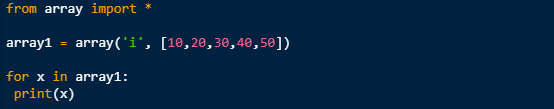Upon executing the above piece of code we get the following output: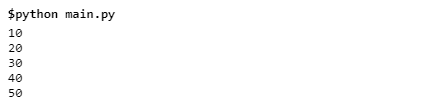## Python Array Index

In order to assess each element in array by using the index of the elements we have to write the following piece of code: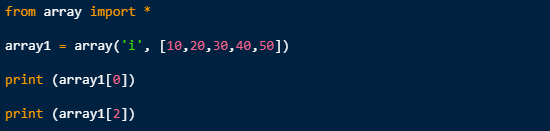As shown in the code we have inserted an element at index position 1. Upon executing the above piece of code, following output is produced.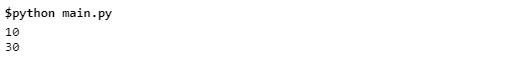## Insert Operation in Python Array

This operation is to insert one or more data elements into an array. We can, on the basis of the requirements, add at the start/beginning, end, or any given index in the array. See the following piece of code and observe how insertion operation is done in Python.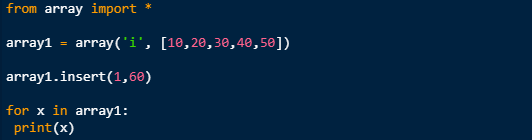Python has a built in insert method and we used it in the above program. With the help of insert () method, we have added the data element at the middle of the array.

Now observe the output of the above piece of code.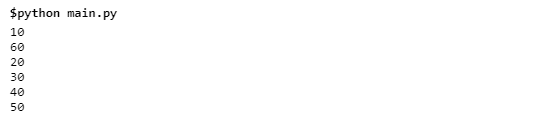When we execute the program, you can see from the output that element is inserted in the array at the index position of 1.

## Delete Operation in Python Array

Delete operation is an easy method in Python to perform. With the help of this in-built method we can remove an existing element from the array and also re-organize them as well.

Look at the following piece of code which is easy as ABC.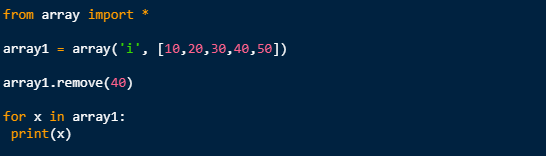Now execute the program and observe the output.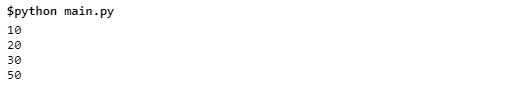You can see number 40 is not available in the list because we have deleted that index from the array.

## Search Operation in Python Array

Search is a built in facility available in Python which helps us search an element in an array based on its index and value. Let us now perform a search operation on array with the following code: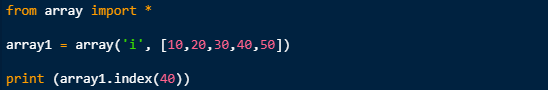Now execute the program, and we get the following result: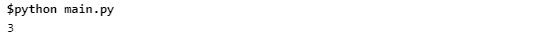When the program is executed, the result shows the index of the element. If there is no value present in the array, then we will get an error by a program.

## Update Operation in Python Array

One of the most important operations in array to manipulate data is Update, which helps us to update an existing element from the array at a given index.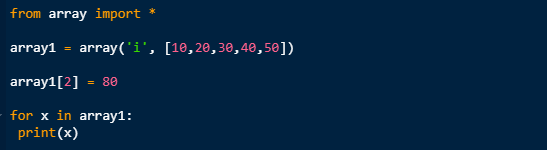In the above piece of code, we simple assign a new value to existing desired index which we wish to update.

When we compile and execute the above program, following input is produced: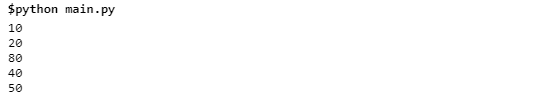As you can observe from the above output, a new value is updated at index 2. As seen, number 30 is now replaced with value 80.

## Negative Indexing in Python Array

This is something which python supports, but many of the programming languages do not. In Python, negative indexing simply means that if we declare index value -1 in the program, then we will get the last element present in the array, and if we declare -2 in the program, then we will get the second to last element present in the array. Look at the simple piece of code below: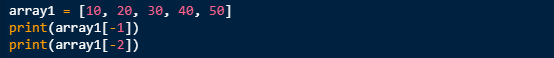Once we compile and run the above code, we get the following output. The last and the second to last element is shown in the output.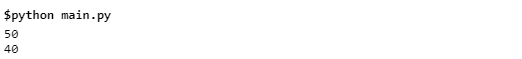## Python Array Length

As we already know that Arrays in Python are just refers to as a lists and finding its length is equivalent to finding a list. Observe the following simple piece of code: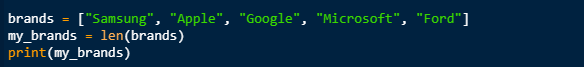Python has built-in len method, which helps in identifying the length of an array. Hence the output is: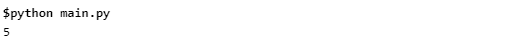## Addition of Element in Python Array

Among other strong and useful built-in methods, another such method is append (). This allows us adding an element to an Array. Look at the following piece of code.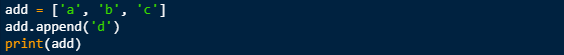As you can see we used the append () method to add ‘d’ in the array by following print () method and passing add object as a parameter. The new element will always add in the end. Hence the output: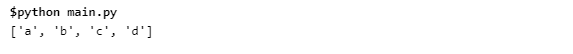## Modify an Element in Python Array

Another useful feature in Python is to modify an element of an array. We can change the values of elements present in the array with the help of indexing and using assignment operator =. In order to modify an element, we have to select the position of any element by using indexing and assignment operator to provide a new value for the element.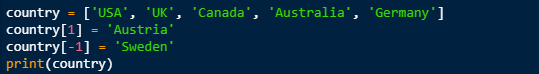As you can observe, that Austria is modified and replaced with UK at index 1, and Sweden is replaced and modified with Germany at last index using -1. As discussed, -1 refers to the last element present in the array. Hence the output is as below: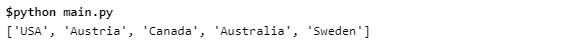## List of Python Array Methods

 Methods Description append() Allows us to add element to the end of the list extend() Allows us to extend all elements of one list to another list insert() Allows us to insert at another index present in the array remove() Allows us to remove an element present in the array at the specified index clear() Allows us to remove all elements from the list index() Allows us to return the index of the first matched element count() Allows us to count number of elements passed as an argument sort() Allows us to sort and element in array in an ascending order by default reverse() Allows us to reverse an order of element in the array copy() Allows us to return a copy of element in the array

## Initialize 2D Array in Python

In the end we will work on two-dimensional array with a simple piece of code. Let’s have a look into it.

All the arrays discussed throughout the topic are one dimensional. In Python, we have a facility to create two-dimensional arrays as well. Two-dimensional arrays are simple define as an array within an array. Let’s have a look into a code as to how an array holds a different array inside it.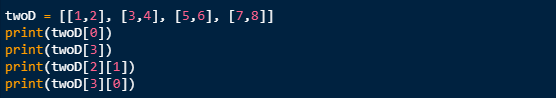When we compile and execute the above piece of good, we get the following output.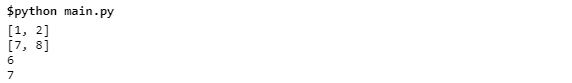As you can see from the above output we have 4 elements and each of them holds another 2 sub-elements.

Happy Learning!